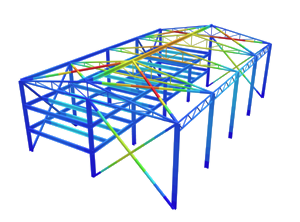SkyCiv Documentation

Your guide to SkyCiv software - tutorials, how-to guides and technical articles

1. Home
2. SkyCiv Connection Design
3. Base Plate Design
4. Design Code – American

# Design Code – American

Reference:

Load : American Society of Civil Engineers, ASCE 7-16
Concrete: American Concrete Institute, ACI 318-19
Steel: American Institute of Steel Construction, AISC 360-16

The Basic load combinations under ASCE 7-16 applies are :

1. $$1.4D$$
2. $$1.2D + 1.6L + 0.5(L_{r} \text{ or } S \text{ or } R)$$
3. $$1.2D + 1.6(Lr \text{ or } S \text{ or } R) + (L \text{ or } 0.5W)$$
4. $$1.2D + 1.0W + L + 0.5(Lr \text{ or } S \text{ or } R)$$
5. $$1.2D + 1.0E + L + 0.2S$$
6. $$0.9D + 1.0W$$
7. $$0.9D + 1.0E$$

where :

$$D$$ = dead load
$$L$$ = live load
$$L_{r}$$ = roof live load
$$S$$ = Snow load
$$R$$ = Rain load
$$E$$ = Earthquake
$$W$$ = Wind load

## Concrete bearing check:

Concrete design bearing strength in compression is designed according to AISC 360-16 Eq. J8-2.

$$F_{b} = \phi _{bearing} \times 0.85 \times f’_{c} \times \sqrt{ \frac{ A_{2} }{ A_{1} } } \leq F_{b, limit} = 1.70 \times f_{c} \times A_{1}$$

where:
$$f’_{c}$$ – concrete compressive strength
$$A_{1}$$ – base plate area in contact with concrete surface
$$A_{2}$$ – concrete supporting surface
$$\phi_{bearing}$$ – resistance factor for concrete ( default value= 0.65 )

## Weld check:

The weld group strength check are in accordance of AISC 360-16 J2

$$(i) R_{n} = R_{nwl} + R_{nwt}$$

or

$$(ii) R_{n} = 0.85R_{nwl} + 1.5R_{nwt}$$

where:

$$R_{nwl}$$ = total nominal strength of longitudinal loaded fillet welds.
$$R_{nwt}$$ = total nominal strength of transversely loaded fillet welds.

## Anchor check:

The Anchor checks applies using code provisions of ACI 318-19 under Chapter 17.

Anchor rods are designed according to AISC 360-16 – J9 and ACI 318-19 – Chapter 17. The following resistances of anchor bolts are evaluated:

• Steel strength of anchor in tension and shear, $$\phi N_{sa}$$ and $$\phi V_{sa}$$.
• Concrete breakout strength in tension and shear, $$\phi N_{cbg}$$ and $$\phi V_{cbg}$$.
• Concrete pullout strength, $$\phi N_{p}$$.
• Concrete side-face blowout strength, $$\phi N_{sb}$$.
• Concrete pryout strength of anchor in shear, $$\phi V_{cp}$$.

#### Steel strength of anchor in tension and shear

Factored steel strength of anchor in tension and shear is determined according to ACI 318-19 – 17.6.1.2 and 17.7.1 as

For Tension

$$\phi _{tension, anc} N_{sa} = \phi _{tension, anc} A_{se,N}f_{uta} \rightarrow$$ equation 17.6.1.2

For Shear

$$\phi _{shear, anc} V_{sa} = \phi _{shear, anc} 0.6A_{se,V}f_{uta} \rightarrow$$ equation 17.7.1.2b

where:

• $$\phi _{tension, anc}$$ – strength reduction factor for anchors in tension ( default value = 0.75 )
• $$\phi _{shear, anc}$$ – strength reduction factor for anchors in shear ( default value = 0.65 )
• $$A_{se,N}$$ – is the effective cross-sectional area of an anchor in tension.
• $$A_{se,V}$$ – is the effective cross-sectional area of an anchor in shear.
• $$f_{uta}$$ – specified tensile strength of anchor steel and shall not be greater than $$1.9f_{ya}$$ and 125 ksi (861.845 Mpa)

#### Concrete breakout strength

Factored concrete breakout strength of anchor in tension and shear is determined according to ACI 318-19 – 17.6.2 and 17.7.1 as

$$\phi N_{cbg} = \phi \frac{ A_{Nc} }{ A_{Nco} } \psi_{ec,N} \psi_{ed,N} \psi_{c,N} \psi_{cp,N} N_{b} \rightarrow$$ equation 17.6.2.ab

where:

$$\phi$$ – strength reduction factor for anchors in tension ( default value = 0.75 ).
$$A_{Nc}$$ – projected concrete failure of a single or group anchors.
$$A_{Nco}$$- project concrete failure area of a single anchor, for calculation of strength in tension if not limited by edge distance or spacing.

$$\psi_{ec,N}$$ – Breakout eccentricity factor in tension.

$$\psi _{ec,N} = \frac{1.00}{ 1 + \frac{e^{‘}_{N}}{1.5 h_{ef}} } \leq 1.00 \rightarrow$$ equation 17.6.2.3.1

$$\psi_{ed,N}$$ – Breakout effect factor in tension.

(a) $$\text{if } C_{a,min} \geq 1.5h_{ef} \text{ then } \psi _{ed,N} = 1.00$$ equation 17.6.2.4.1a

and

(b) $$\text{if } C_{a,min} < 1.5h_{ef} \text{ then } \psi _{ed,N} = 0.70 + 0.3\frac{C_{a,min}}{1.5h_{ef}}$$ equation 17.6.2.4.1b

$$\psi_{c,N}$$ – Breakout cracking factor in tension.

$$\psi _{c,N} = 1.25$$ for cast-in anchors

$$\psi_{cp,N}$$ – Breakout splitting factor in tension.

(a) $$\text{if } C_{a,min} \geq C_{ac} \text{ then } \psi _{cp,N} = 1.00$$ equation 17.6.2.4.1a

and

(b) $$\text{if } C_{a,min} < C_{ac} \text{ then } \psi _{cp,N} = \frac{ C_{a,min} }{ C_{ac}} \geq \frac{ 1.5h_{ef} }{ C_{ac} }$$ equation 17.6.2.4.1b

$$N_{b}$$ – basic concrete breakout strength in tension of a single anchor in cracked concrete.

#### Concrete pullout strength

Factored concrete pullout strength of an anchor is defined in ACI 318-19 – 17.6.3 as

ϕNpn = ϕΨc,P Np

where:

$$\phi$$ – strength reduction factor for anchors in tension ( default value = 0.70 ).
$$\psi _{c, P}$$ – modification factor for concrete condition

For cracked concrete:

$$\psi _{c, P}$$ = 1.0

For non-cracked concrete:

$$\psi _{c, P}$$ = 1.4

$$N_{p}$$ – Anchor pullout strength

For cracked concrete:

$$N_{p} = 8A_{brg}f^{‘}_{c}$$ equation 17.6.3.2.2a

For non-cracked concrete:

$$N_{p} = 0.9f^{‘}_{c}e_{h}d_{a} \rightarrow$$ equation 17.6.2.2.b

where $$3d_{a} \leq e_{h} \leq 4.5d_{a}$$

$$f^{‘}_{c}$$ – specified compressive strength of concrete.
$$A_{brg}$$ – net bearing area of the head of stud, anchor bolt or headed deformed bar.
$$e_{h}$$ – distance from the inner surface of the shaft of a J-bolt or L-bolt to the outer tip of the J- or L-bolt.
$$d_{a}$$ – outside diameter of anchor or shaft diameter of headed stud, headed bolt, or hooked bolt.

#### Concrete side-face blowout strength

Factored concrete side-face blowout strength of an anchor is defined in ACI 318-19 – 17.6.4 as

$$\phi N_{sb} = 160C_{a1}\sqrt{A_{brg}}\lambda _{a} \sqrt{f^{‘}_{c} } \rightarrow$$ equation 17.6.4.1

where:

$$f^{‘}_{c}$$ – specified compressive strength of concrete.
$$A_{brg}$$ – net bearing area of the head of stud, anchor bolt or headed deformed bar.
$$\lambda_{a}$$ – modification factor to reflect the reduced mechanical properties of lightweight concrete in certain concrete anchorage application.